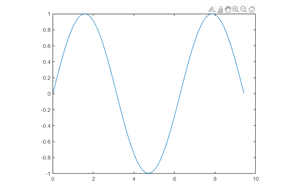# How to create a function in MATLAB ?

• Last Updated : 26 May, 2021

A function is a block of statements that intend to perform a specific task. Functions allow the users to reuse the code frequently. MATLAB has several predefined functions which are ready to use such as sin(), fact(), cos() etc. MATLAB also allows the users to define their own functions.

Syntax:

function output_params = function_name(iput_params)

% Statements

end

• The function starts with the keyword function.
• Returning variables of the function are defined in output_params
• function_name specifies the name of the function
• input_params are input arguments to the function

Below are some examples that depict how to use functions in MATLAB:

Example 1: Function with one output

The function calculates the mean of the input vector

## Matlab

 `% Input vector``values = [12, 4, 8.9, 6, 3];` `% function return mean of vector c``function` `m = stat(x)``    ``n = length(x);``    ``m = sum(x)/n;``end` `mean = stat(values)`

Output :

`mean = 6.7800`

Example 2: Function with multiple outputs

The function calculates both nCr and nPr of inputs n and r.

## Matlab

 `% Input``x = 3;``y = 2;` `% Function return p = nPr and c = nCr``function` `[p,c] = perm(n,r)``    ``p = factorial(n)/factorial(n-r);``    ``c = p*factorial(r);``end` `[p,c] = perm(x,y)`

Output :

```p = 6
c = 12```

Example 3: Multiple functions in a file

• stat2() function calculates the standard deviation of the input vector.
• stat1() calculates the mean of the input vector.

## Matlab

 `values = [12, 4, 8.9, 6, 3];` `% Function returns standard deviation of vector x``function` `sd = stat2(x)``    ``m = stat1(x);``    ``n = length(x)``    ``sd = sqrt(sum((x-m).^2/n));``end` `% Function returns mean of vector x``function` `m = stat1(x)``    ``n = length(x);``    ``m = sum(x)/n;``end` `stat2(values)`

Output :

```n = 5
ans = 3.2975```

Example 4: Function with no input_params

In this program, we will create the sin_plot() function that plots the sin() function

## Matlab

 `% Plotting sin(x) function``function` `sin_plot()``    ``x = linspace(0,2*pi,100);``      ``y = sin(x);``    ``plot(x,y);``end` `sin_plot()`

Output :My Personal Notes arrow_drop_up